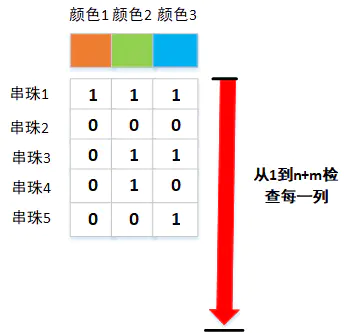• 累计撰写 270 篇文章
• 累计创建 6 个标签
• 累计收到 1 条评论

### 目 录CONTENT# 字节--手串.md2021-08-22 / 0 评论 / 0 点赞 / 6 阅读 / 2,105 字 / 正在检测是否收录...

# 字节--手串

@[toc]

## 一、题目描述

•  输入描述:
第一行输入n，m，c三个数，用空格隔开。(1 <= n <= 10000, 1 <= m <= 1000,
1 <= c <= 50) 接下来n行每行的第一个数num_i(0 <= num_i <= c)表示
第i颗珠子有多少种颜色。接下来依次读入num_i个数字，每个数字x表示第i颗
柱子上包含第x种颜色(1 <= x <= c)

•  输出描述:
一个非负整数，表示该手链上有多少种颜色不符需求。

5 2 3
3 1 2 3
0
2 2 3
1 2
1 3

2



## 二、分析

•
1. 首先输入了珠子个数n 要求连续珠串不能重复的个数是m，串珠颜色有c种
•
1. 这道题也是需要设计一个良好的数据结构才能求解
•
1. 我们可以把这个串珠和颜色设计为一个二维矩阵，行向量是每一个串珠，列向量是串珠颜色，列向量总数为c，如果在i行j列上的这个数对应的串珠的位置没有这个颜色那么置0
•  简言之，就是将每个串珠的颜色都扩充为一样的c种，原本拥有的就为1，没有的就为0，那么就可以设计为一个0-1表示的二维矩阵，如下图中所示•  4.其次，再研究研究输出结果，是要求不满足m个串珠连续的颜色总和，那么这个问题就转变为了我对每种颜色检查是否符合结果
•  5. 针对每一种颜色检查的是否有连续个m个串珠针对同一种颜色同时出现了两个1（针对矩阵而言
•  因为需要针对同一种颜色从第一个串珠（第一行）检查到最后一个串珠(最后一行)，那么整体的遍历结束就会结束于n + m（因为到达最后一行相邻的串珠是第一行开始到m-1）
•
1. 依次检查每一列是否符合要求即可得到最后的总数

## 三、代码

#include<iostream>
#include<vector>
#include<unordered_set>

using namespace std;
int main()
{
int n;
int m;
int c;
cin>>n>>m>>c;

//构建二维数组
//行代表珠串数
//列代表颜色
//0代表该珠串没有出现某颜色，1代表出现某颜色
vector<vector<int>> matrix(n,vector<int>(c));
for (int i = 0; i < n; i++)
{
int total ;
cin>>total;
for (int j = 0; j < total; j++)
{
int k ;
cin>>k;
matrix[i][k - 1] = 1;
}
}

//构造结果数组
//如果对应颜色处为1代表该颜色违规
//用数组保存的原因是可能出现一个颜色多次违规的情况
vector<int> result(c);

//遍历所有颜色，也可以理解为遍历数组只不过是遍历每一颜色列，纵向遍历
for (int i = 0; i < c; i++)
{
//标记某一颜色上一次出现的位置
int lastIndex = -1;

//end为珠串判断结束的位置，等于n + m - 1是因为珠串是环形
int end = n + m - 1;
for (int j = 0; j < end; j++)
{
//防止越界
int row = j % n;
//如果等于1就代表该位置出现i号颜色，那么就需要和上一次出现i号颜色进行判断
if(matrix[row][i] == 1)
{
//如果上一次出现该颜色的位置为-1，就代表该颜色是第一次出现
//进行标记
if (lastIndex == -1)
lastIndex = j;
else
{
//反之就代表该颜色是多次出现，就需要判断其上一次出现的位置和
//当前出现的位置之间的距离是否超过m
//超过m就代表该颜色违规，进行标记
if (j - lastIndex < m)
result[i] = 1;
//反之就代表该颜色多次出现的距离超过m，就更新lastIndex即可
else
{
lastIndex = j;
}
}
}
}
}
int sum = 0;
for (int i = 0; i < c; i++)
{
if (result[i] == 1)
sum++;
}
cout<<sum<<endl;
return 0;
}


0